CS348b Final Project: Fractal Primitives

Daniel Wong

Overview

The purpose of this project was to create an interface to explore fractal objects. I started out with the intent of rendering 3D Julia sets, but later tried to generalize the interface wherever possible to apply to as many fractals as possible. In ray-tracing fractals, two problems present themselves: how to intersect a ray with the set, and finding the normal of the set at a particular point. Click on any of the pictures for the original .tiff file, especially the above picture, which looks incredible at 1600x1200.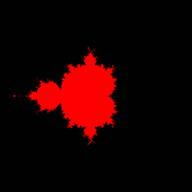Intersections

The first approach I took was to simply evaluate points along the ray until I found a point within the set. With this approach, I was able to determine whether or not I was evaluating points correctly. Here’s an image of the 2D Mandelbrot set that’s been extruded a small distance towards the camera.

There are 3 problems that need to be addressed with this approach: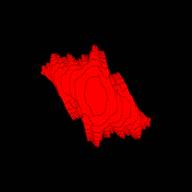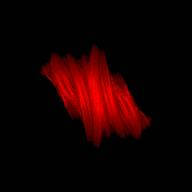Z-Resolution:

With step sizes that are too large, you run the risk of getting steps in the fractal instead of smooth gradations. To the left is an example of this effect, with the complete fractal shown for comparison.

Thin structures:

Even with smaller step sizes, the new risk is that thin structures of the fractal may lie between two sample points. I was unable to solve this problem, except by decreasing the step sizes further, which leads to:

Speed:

Obviously, taking a huge number of small steps on rays that never intersect the set can be very time consuming. The traditional method of speeding this up is to use functions that approach some value as you approach the boundary of the set, such as Distance Estimators or the Newton-Raphson method. The problem is that these functions need to be pre-computed for various types of 3D fractals. I had success in implementing the Distance Estimator for 3D Julia sets, but not for other types of fractals (see below).

Normals

As with Distance Estimators, normals can be explicitly determined from certain types of 3D fractals. I had originally written code to handle the explicit case of 3D quadric Julia sets, but later decided to generalize the code to calculate normals for generic fractals. After determining the point of intersection, I shoot two more rays that are perturbed slightly (based on the distance to the intersection point) to obtain two tangents. I had such great success with this method that I even used it for the 3D quadric Julia sets.

Other Images

Another 3D quadric Julia set, with a zoomed in portion on the right (w/ different lighting conditions)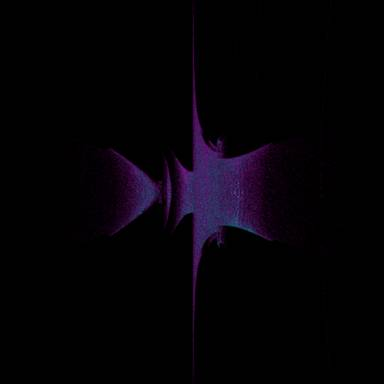A cubic Julia set. The set is revolved around the horizontal axis. The set number of iterations was set slightly high, which causes the “noise” on the surface,  since higher iterations cause the fractals to become rougher.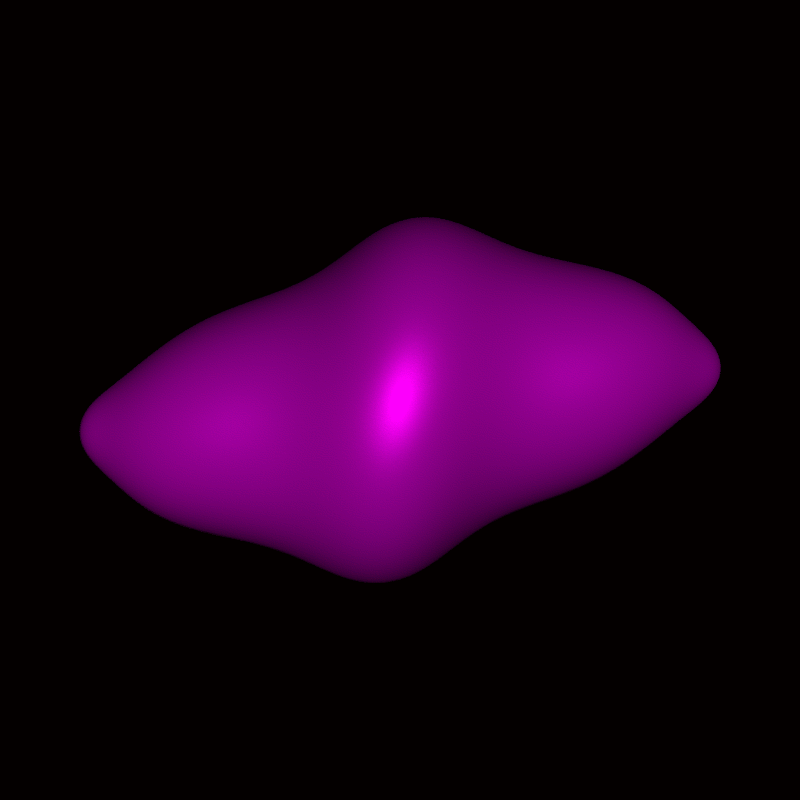An animation, where the number of iterations is varied.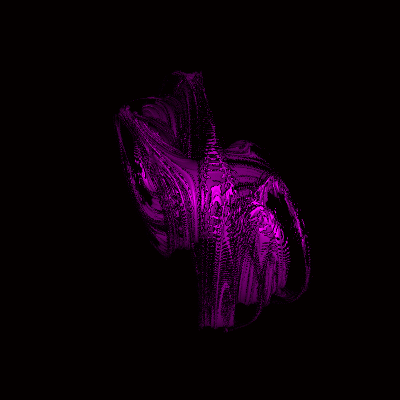Another 3D quadric Julia set, rotated.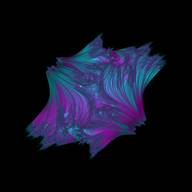The 3D Mandelbrot set. A 2D Julia Set with the real part of c varying in the z-direction. A 2D Julia set and a 2D Mandelbrot set, given a small amount of thickness.